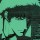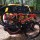## Introduction to memoization with Javascript

Memoization is a function optimization technique used to avoid remaking calculations in subsequent function calls.  The classic example, which we’ll demonstrate here, is the factorial function.  5! = 5 * 4 * 3 * 2 * 1… factorials are recursive in nature if we give it some thought.  5! = 5 * 4! and 4! = 4 * 3! and so on.  By recognizing this pattern, we can also recognize that if we calculate 5! and save the results, then we should not have to recalculate it when we do 7!.  Instead of calculating 7! by 7 * 6 * 5 * 4 * 3 * 2 * 1, we’ll calculate it by 7 * 6 * 5!.

We’ll first examine the naive approach and then the memoization method.  All in Javascript, and all with code samples…

### The Naive Approach

The naive approach is very simple.  This is a snip / modification of the larger script, which you can see at the bottom of this post, but you will get the point.

```function naive(n) {
if(n <= 1) { return 1; }
return n * naive(n - 1);
}
}```

In this function, the parameter n is the value of the factorial we wish to calculate.  In this recursive function call, our base case will be when the parameter is 1.  1! = 1, of course, so instead of recursing we’ll simple return the value.  If n is greater than 1, we’ll recursively call the function, returning the current value we wish to calculate multiplied by n – 1.

While this approach works, it is wasteful.  By calling naive(5), we calculate 5 * 4 * 3 * 2 * 1.  Instead of saving the value of naive(5) to be used the next time we call the function, we effectively discard it.  When we call, naive(7), we cannot reuse the value we found for naive(5).

### The Memoization Approach

The memoization approach is only slightly more complicated.  Again, this code is a section of a larger script, so bear with me.

```var lookup = {};
function memoized(n) {
if(n <= 1) { return 1; }
if(lookup[n]) {
return lookup[n];
}
lookup[n] = n * memoized(n - 1);
return lookup[n];
}```

First, we create an empty object to serve as a lookup table for our factorial function.  This is where we’ll save the factorials that we’ve already calculated.  Our function has the same parameter and base case as our naive example.  The difference in the function is the lookup.  If the value of n is a key in our lookup table, then we simply return that value.  If the value isn’t in the lookup table, we’ll save it in the lookup table for later.  Now that we’ve found and saved the value of factorial n, we’ll return it to the caller.

If we first call memoized(5), we’ll calculate 5 * 4 * 3 * 2 * 1.  If we then call memoized(7), we’ll calculate 7 * 6 * 5!, the 5! being saved in the lookup table.  Much faster!

### The Full Script and Observations

I said previously that the snippets above were part of a larger script.  In the process of writing this post, I wrote the functions as part of a Javascript object I also wrote a function to test the speed increase.  While not absolutely scientific, it should help you grasp the kind of increase you can expect over the succession of function calls.  The script was written and executed in node.js, but should work just as well in Firebug or even Rhino if you remove the #! script declaration.

For what it’s worth, on my machine node.js executed the test set for the naive method in ~5ms and the memoization method in ~1ms.  Firebug / Firefox 6.0 executed the test set for the naive method in ~14ms and the memoization method in ~1ms.  I think that says something about the speed of the V8 Javascript engine.

Feel free to use / modify this script for your own tests.  I hope that this example helps you speed up your code in the future.

```#!/usr/local/bin/node
var factorial = (function() {
var lookup = {};
return {
//show_lookup is mainly for debugging purposes
show_lookup : function() { return lookup; },
run : {
naive : function(n) {
if(n <= 1) { return 1; }
return n * this.naive(n - 1);
},
memoized : function(n) {
if(n <= 1) { return 1; }
if(lookup[n]) {
return lookup[n];
}
lookup[n] = n * this.memoized(n - 1);
return lookup[n];
}
}
};
})();
var caller = function(method) {
var start = (new Date()).getTime();
for(var i = 0; i <= 150; i++) {
factorial.run[method](i);
}
for(var j = 150; j > 1; j--) {
factorial.run[method](j);
}
return (new Date()).getTime() - start;
};
console.log(caller("naive"));
console.log(caller("memoized"));```

1.Daniel Fitzpatrick

Really what you should do is write a function which takes a pure function as an argument and spits out a memoized version of the function. Ought to be relatively simple, since all you need to do is check a hashmap for a value, and call the pure function if it’s not there, storing the value prior to returning it.

2.Daniel Fitzpatrick

Also, once you get that written, you should revisit the collatz conjecture and see how high you can get with bigints

3.Carlos Roque

So in a real world escenarion, the script would need to be running for a long period and at the same time be used multiple times to collect data. otherwise if the look up table has to be populated over and over again, are you really seeing any performance inprovements? would you consider storing the lookup table as a way to improve on your script?

4. In the example I gave, yes, the function has amortized speed increases; there are no useful keys in the look-up table until the function has been fully executed at least once. However, this has to do with the linearity of the factorial function (5! * 4! * 3!…).

Consider the Fibonacci sequence where f(n) = f(n – 1) + f(n – 2). Given n = 6 on the first call to the function, fib(5) and fib(4) will be calculated. However, fib(5) will also calculate fib(5-1), which has already been calculated by fib(6). That is wasted effort. If you run this script in Firebug, it will take a long time to complete or freeze/crash.

```var fib = function(n) {
if(n == 0 || n == 1) {
return n;
}
return fib(n - 1) + fib(n - 2);
}
fib(100);
```

However, this snippet of code will run very quickly. The function is not amortized; it will run quickly on the first execution.

```var computed = {};
var fib = function(n) {
if(n == 0 || n == 1) {
return n;
}
if(computed[n]) {
return computed[n];
}
var val = fib(n - 1) + fib(n - 2);
computed[n] = val;
return val;
}
fib(100);
```

As for storing the look-up table in a more persistent location… that is definitely a possibility and a good idea depending on the context. I believe there is another term for this other than memoization, but I can’t think of it right now ?

5.bj99

its even more impressive if you tune the numbers a bit ?
``` [...] var caller = function(method) { var start = (new Date()).getTime(); for(var i = 0; i <= 15000; i++) { factorial.run[method](i); }```

``` ```

``` for(var j = 15000; j > 1; j–) { factorial.run[method](j); } return (new Date()).getTime() – start; }; console.log(caller(“naive”)); console.log(caller(“memoized”)); ```
naive: 141477
memoized: 40